# 6-1输出月份英文名#

## 流程图##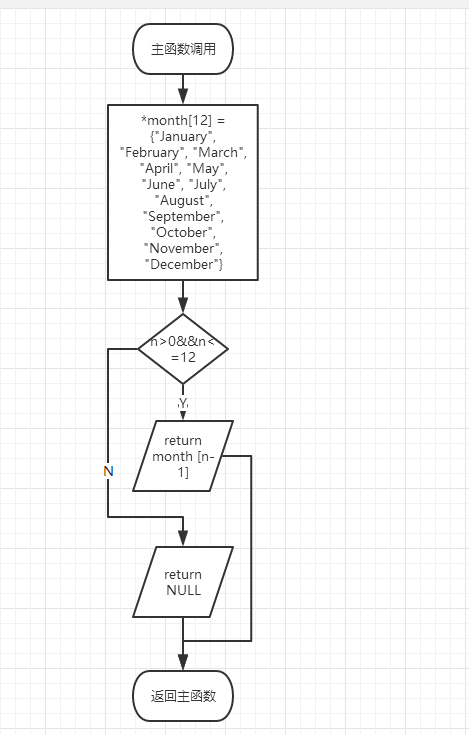## 实验代码##

char *getmonth( int n )
{
char *month = {"January", "February", "March", "April", "May", "June", "July", "August", "September", "October", "November", "December"};
if(n>0&&n<=12)
{
return month [n-1];}
else
return NULL;
}


# 6-2查找星期#

## 流程图##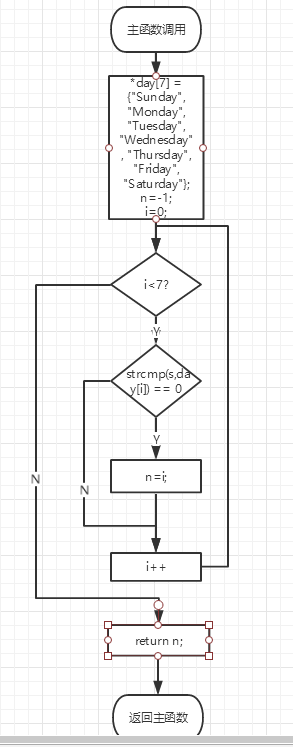## 实验代码##

int getindex( char *s )
{
char *day = {"Sunday", "Monday", "Tuesday", "Wednesday", "Thursday", "Friday", "Saturday"};
int n=-1;
int i=0;
for(i=0; i<7; i++)
{
if( strcmp(s,day[i]) == 0 )
n=i;

}
return n;
}


# 6-3 计算最长的字符串长度#

## 实验代码##

	int max_len( char *s[], int n )
{
int i,max=0,t=0;
max=strlen(s[i]);
for(i=1;i<n;i++)
{
t=strlen(s[i]);
if(max<t)
max=t;
}
return max;
}


## 错误信息##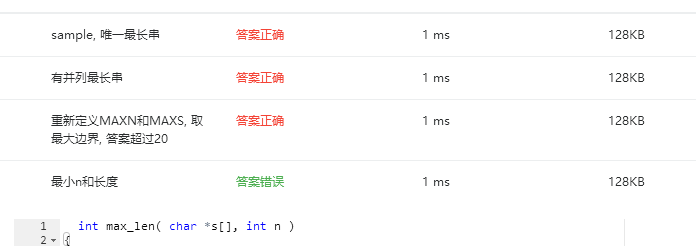# 6-4 指定位置输出字符串#

## 实验代码##

char *match( char *s, char ch1, char ch2 ){

int i=0,j=0,k=0,len=0;
char *p=NULL;

for(i=0;i<len;i++){
if(s[i]==ch1){
p=&s[i];
for(j=i;j<len;j++){
if(s[j]!=ch2){
printf("%c", s[j]);
}
if(s[j]==ch2){
printf("%c\n", s[j]);
return p;
}
}
printf("\n");
return p;
}
}
printf("\n");
return p;
}


## 错误信息### 编程题#

有一个axb的数组,该数组里面顺序存放了从1到a*b的数字。其中a是你大学号的前三位数字，b是你大学号的后四位数字，比如你的学号是2017023936，那么数组大小是201 x 3936，数组中顺序存放了1到791136（201和3936的积）的整数. 要求用筛选法,把该数组里的质数找出并打印出来，打印格式为5个质数一行，数字间用空格隔开。



## 实验代码##

#include <stdio.h>
#include <math.h>
int main ()
{
int n=0,i=0,j=0,x=0;
n = 201*990;
int axb[n];

for(i=1;i<n;i++)
{
axb[i-1] = i;
}
for(i=1;i<=n;i++)
{
for(j=2;j<=sqrt(i);j++)
{
if(i%j==0){
axb[i-1]=1;
}
}
}
for(i=0,x=0;i<n;i++)
{
if(axb[i]!=1){
printf("%d ",axb[i]);
x++;
if(x%5 == 0){
printf("\n");
}
}
}
return 0;
}


# 学习总结和进度#

## 1、总结两周里所学的知识点，回答下列问题？（用自己的话表达出你的理解，网上复制粘贴没有分数）（5分）

 （1）如何理解指针数组，它与指针、数组有何关系？为何可以用二级指针对指针数组进行操作？

（2）将C高级第三次PTA作业（1）任何一个题目改为使用二级指针对指针数组进行操作。

（3）用指针数组处理多个字符串有何优势？可以直接输入多个字符串给未初始化的指针数组吗？为什么？##


（1）指针数组能更方便的达到我们的要求。因为指针数组可以被指向地址。
(3)可以减少运算时间和内存占用。可以。

## 我的GIT##

[地址]https://coding.net/u/baisongtao/p/baisongtao/git/tree/master/?public=true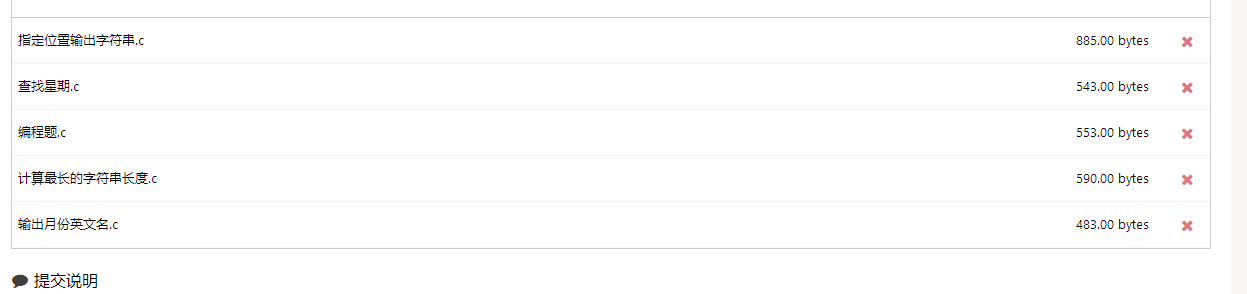## 记录表##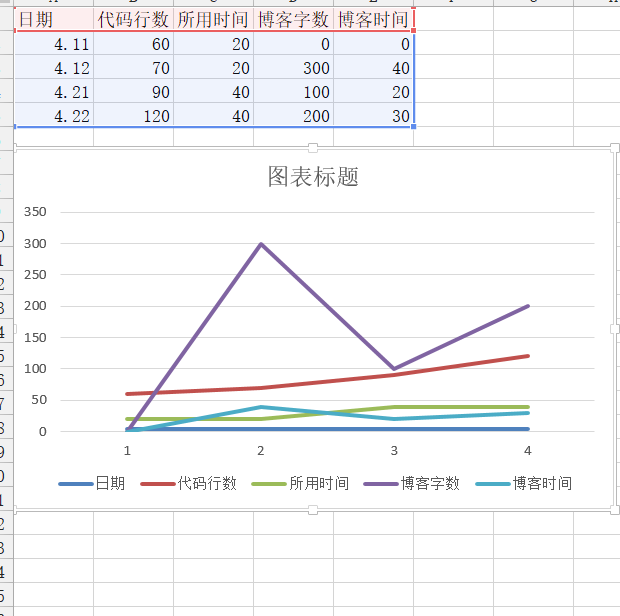posted on 2018-04-15 17:08  白松涛  阅读(229)  评论(2编辑  收藏  举报